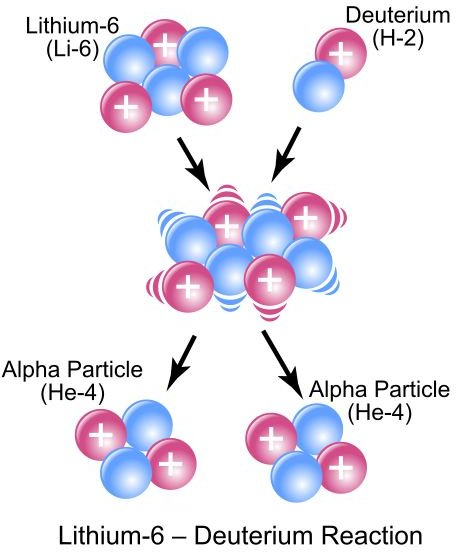# Learn the Differences between Nuclear and Chemical Reactions: Explanation

## Nuclear vs Chemical Reactions

What is the difference between a nuclear and a chemical reaction? As it turns out, this is pretty simple. In a chemical reaction, the bonds between elements are broken and reformed to create new molecules that contain the same elements. For example, in the chemical equation 2H2 + O2 = 2H2O, we now have a new substance – water – but the underlying elements are the same.

In a nuclear reaction, on the other hand, the nucleus of the element changes to make a new element. For example, bombarding lithium with neutrons causes the creation of tritium, a radioactive isotope of hydrogen which is extremely rare in nature.

Stated another way, a chemical reaction involves an atom’s electrons – bonds are broken and restored in the search for a more stable configuration – while a nuclear reaction involves the atom’s nucleus.

## Energy

Another difference is the amount of energy required. While a chemical reaction requires or gives off a relatively small amount of energy through the breaking and forming of atomic bonds, a nuclear reaction gives off a large amount of energy through the splitting (fission) or fusing (fision) of atomic neclei.

## Decay of Radioactive Elements

As with chemical reactions, not all nuclear reactions are man-made. A radioactive isotope randomly loses energy in the form of alpha particles, decaying into atoms that may be of a different type. For example, a carbon-14 atom may decay into a nitrogen-14 atom. In some cases, the atom remains the same element, but at a lower energy state.Although the amount of time it takes for a given atom to give off an alpha particle is random, a large number of such atoms will give off particles at a predictable rate. This rate is known as the half-life, and is the amount of time it takes for half of the atoms to decay; the half-life can be trillions of years or a tiny fraction of a second. For example, Carbon-14 has a half-life of 5730 years , which means that if you start with a certain amount of C14, after 5730 years or so you should have half as much, then after 11,460 years or so you should have a quarter of the amount you started with, and so on. This reaction is used in carbon dating: the ratio of carbon-14 to carbon-12 in a living organism is know, but when something dies, the decaying carbon-14 is no longer replaced; as a result, we can estimate the age of a formerly living organism by taking a sample and comparing the relative amounts of C12 and C14.

## Similarities

How are these two reactions similar? In both cases, we can predict how elements will combine by writing balanced equations; because matter and energy are neither created nor destroyed, we know that what we put into the system will be the same as what we get out, albeit possibly in a changed form. Both types of reactions are, of course, very important for our life here on earth!